复杂 Gremlin 查询的调试方法Tom(⊙o⊙)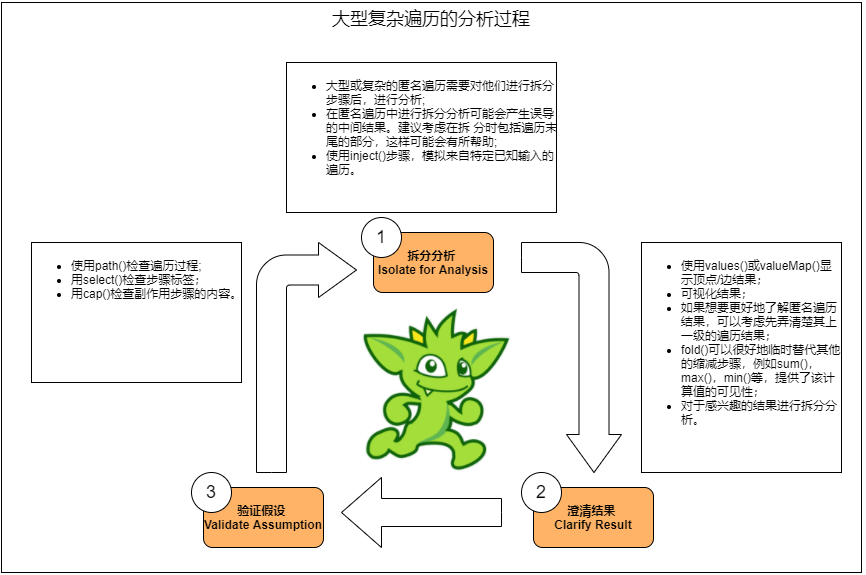1. Gremlin 简介

Gremlin 是Apache TinkerPop 框架下的图遍历语言。Gremlin 是一种函数式数据流语言，可以使得用户使用简洁的方式表述复杂的属性图（property graph）的遍历或查询。每个 Gremlin 遍历由一系列步骤（可以存在嵌套）组成，每一步都在数据流（data stream）上执行一个原子操作。

Gremlin 是一种用于描述属性图中行走的语言。图形遍历分两个步骤进行。

1.1. 遍历源（TraversalSource）

• GraphTraversalSource.V（Object ... ids）：从图形的顶点开始遍历（如果未提供 id，则为所有顶点）。

• GraphTraversalSource.E（Object ... ids）：从图形的边缘开始遍历（如果未提供 id，则为所有边）。

1.2. 图遍历（GraphTraversal）

V()和 E()的返回类型是 GraphTraversal。 GraphTraversal 维护许多返回 GraphTraversal 的方法。GraphTraversal 支持功能组合。 GraphTraversal 的每种方法都称为一个步骤(step)，并且每个步骤都以五种常规方式之一调制(modulates)前一步骤的结果。

1. map：将传入的遍历对象转换为另一个对象（S→E）。

2. flatMap：将传入的遍历对象转换为其他对象的迭代器（）。

3. filter：允许或禁止遍历器进行下一步（S→S∪∅）。

4. sideEffect：允许遍历器保持不变，但在过程中产生一些计算上的副作用（S↬S）。

5. branch：拆分遍历器并将其发送到遍历中的任意位置（S→{$S1→E^，…，S_n→E^$}→E*）。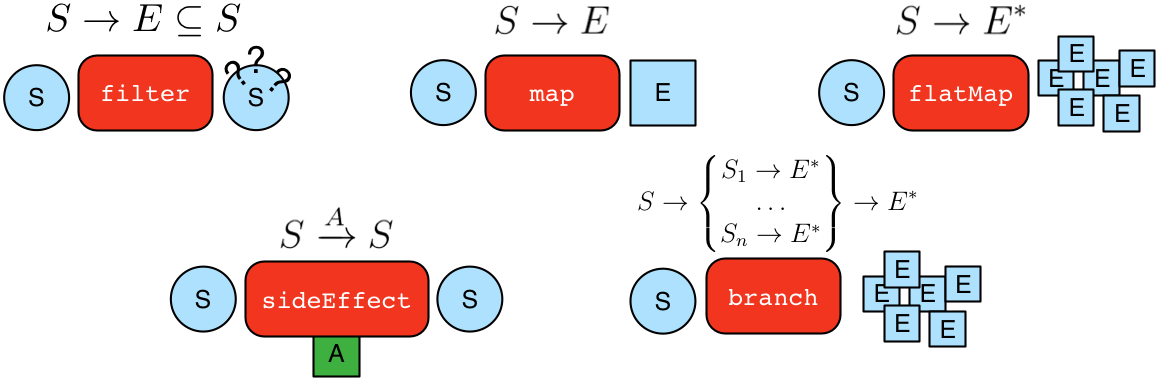GraphTraversal 中几乎每个步骤都从 MapStep，FlatMapStep，FilterStep，SideEffectStep 或 BranchStep 扩展得到。

• 举例：找到 makro 认识的人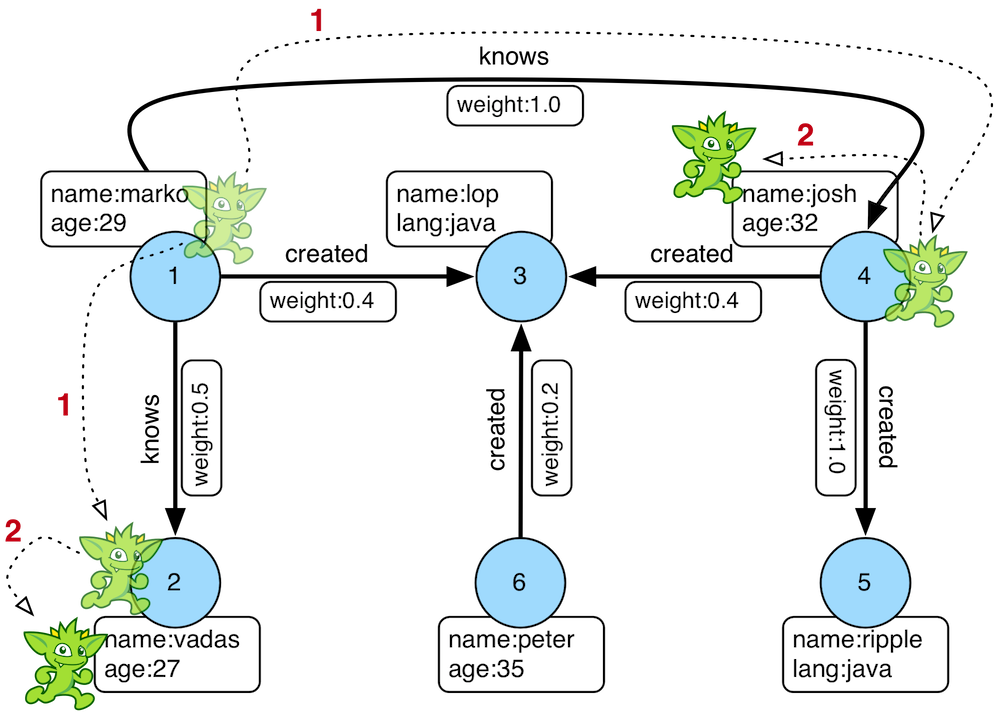2. 复杂 Gremlin 查询的调试

Gremlin 的查询都是由简单的查询组合成复杂的查询。所以对于复杂 Gremlin 查询可以分为以下三个步骤，并逐步迭代完成所有语句的验证，此方法同样适用编写复杂的 Gremlin 查询。

2.1. 迭代步骤

1. 拆分分析步骤，划大为小，逐步求证；

2. 输出分步骤的结果，明确步骤的具体输出内容；

3. 对输出结果进行推导和检验；扩大分析步骤，回到步骤 1 继续，直到清楚所有结果。2. 用例

2.2.1. 图结构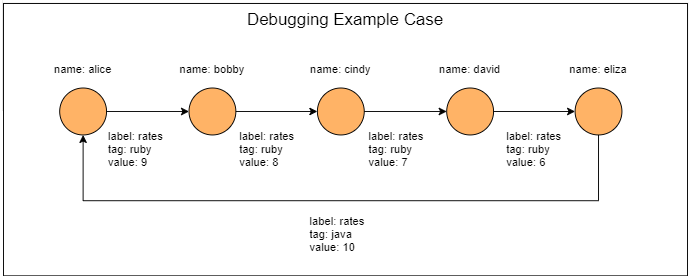2.2.2. 查询语句

gremlin>g.V().has('name','alice').as('v').   repeat(outE().as('e').inV().as('v')).     until(has('name','alice')).   store('a').     by('name').   store('a').     by(select(all, 'v').unfold().values('name').fold()).   store('a').     by(select(all, 'e').unfold().        store('x').          by(union(values('value'), select('x').count(local)).fold()).        cap('x').        store('a').by(unfold().limit(local, 1).fold()).unfold().        sack(assign).by(constant(1d)).        sack(div).by(union(constant(1d),tail(local, 1)).sum()).        sack(mult).by(limit(local, 1)).        sack().sum()).   cap('a')==>[alice,[alice,bobby,cindy,david,eliza,alice],[9,8,7,6,10],18.833333333333332]

2.3. 调试过程

1. 拆分查询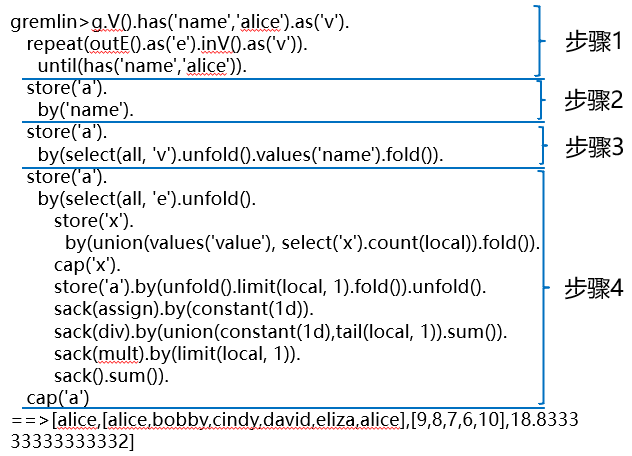• 执行第一部分步骤

gremlin> g.V().has('name','alice').as('v').......1> repeat(outE().as('e').inV().as('v')).......2> until(has('name','alice'))==>v

1. 澄清结果

gremlin> g.V().has('name','alice').as('v').......1> repeat(outE().as('e').inV().as('v')).......2> until(has('name','alice')).valueMap()==>[name:[alice]]

1. 验证假设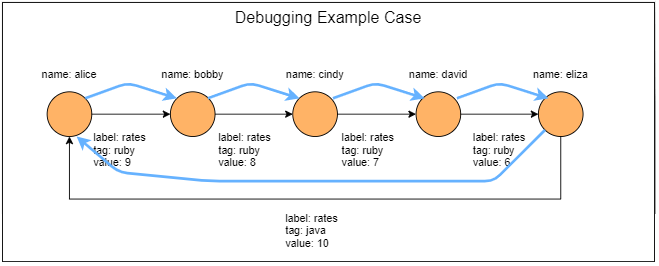1. 使用 path(), 验证推导过程

g.V().has('name','alice').as('v').......1> repeat(outE().as('e').inV().as('v')).......2> until(has('name','alice')).path().next()==>v==>e[0-rates->2]==>v==>e[2-rates->4]==>v==>e[4-rates->6]==>v==>e[6-rates->8]==>v==>e[8-rates->0]==>v

• 输出结果与推导结果一致，扩大查询语句, 回到步骤 1;

• 如不一致或不理解结果, 缩小步骤范围, 可以采用此步骤的上一层查询步骤,回到步骤 1;

gremlin> g.V().has('name','alice').as('v').......1> repeat(outE().as('e').inV().as('v')).......2> until(has('name','alice')).......3> store('a').by('name')==>v

• 如此循环直到完全理解整个查询。

3. 总结

• 在分析的过程，采用划分查询语句的方法，分步理解，采用漏斗式的方法，逐步扩大对语句的理解；

对每步的查询结果，可以采用利用 valueMap(), path(), select(), as(), cap() 等函数输出和验证结果；

对于不清楚结果的步骤或与期望值不一致，缩小查询步骤,可以采用输出步骤的前一步骤作为输出点，进行输出和验证；

对于上一层数据的结果明确的情况下，可以采用 inject()方式注入上层输出，继续后续的输出和验证；

要注意步骤最后的函数对整个数出结果的影响。

4. 参考评论